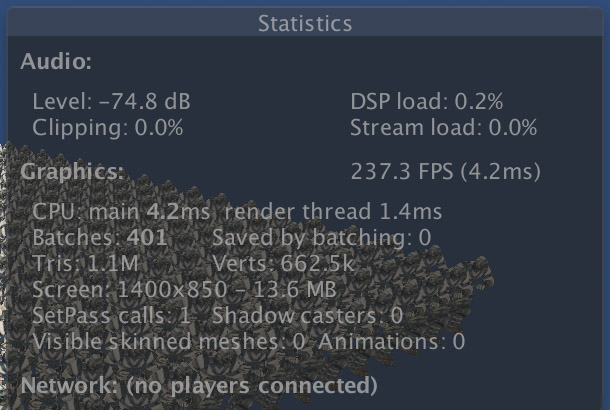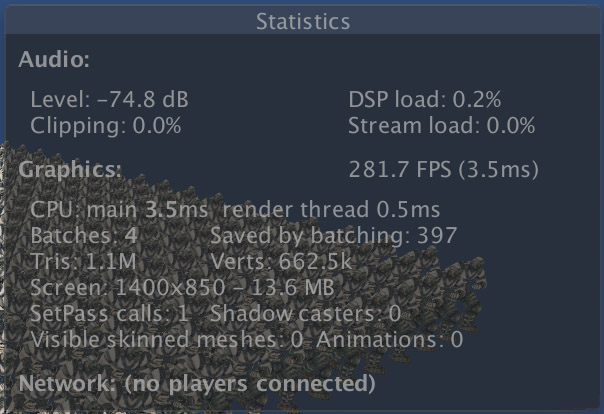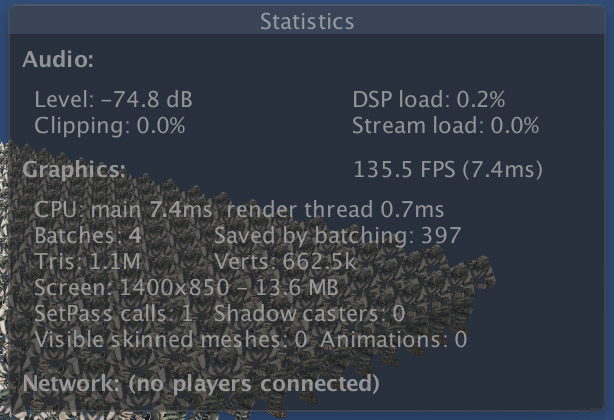# Jim's GameDev Blog# GPU Instancing

2016-12-4``````// App Code
// renderers 数组里存储的是每个模型的 MeshRenderer
MaterialPropertyBlock mtrlPropBlock = new MaterialPropertyBlock();
int numRenderers = renderers.Length;
for(int i = 0; i < numRenderers; ++i)
{
Vector3 modelPos = renderers[i].transform.position;
renderers[i].SetPropertyBlock(mtrlPropBlock.SetVector);
}

// 这一步是蒙皮操作，没有任何变化
float4 pos =
mul(_Matrices[v.tangent.x], v.vertex) * v.tangent.y +
mul(_Matrices[v.tangent.z], v.vertex) * v.tangent.w;
// 原来这里是直接和 MVP 矩阵相乘，现在改为世界坐标系下的偏移
o.vertex = mul(unity_ObjectToWorld, pos);
o.vertex.xyz += UNITY_ACCESS_INSTANCED_PROP(_ModelPos).xyz;
o.vertex = mul(UNITY_MATRIX_VP, o.vertex);
``````